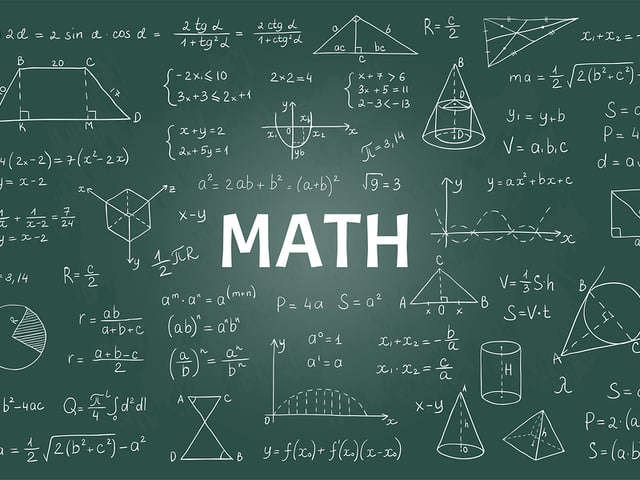# What Level of Math Is Tested on the PSAT/NMSQT®?

The Preliminary Scholastic Achievement Test® (PSAT)/National Merit Scholarship Qualifying Test® (NMSQT) is a nationwide standardized test designed for juniors in high school. It is used both as a college entrance examination, and to determine eligibility for National Merit Scholarships. It is a timed test and is divided into three sections: Writing and Language, Reading, and Math.

## What to Know about the PSAT/NMSQT Math Section

The total time for the test is two hours and 45 minutes. The time allocated to the math portion is 70 minutes. Because the test is designed for juniors in high school, questions require knowledge of algebra I and geometry. There are 48 math questions; most of these will be multiple-choice, with four possible choices. However, for some questions, you will be required to solve the problem and enter your answer on the grid provided.

You will be allowed to use an approved calculator on a portion of the test. Check what types are approved prior to the test. The test is only given at designated sites. Your abilities to reason and analyze information are key to success on the test.

## What Areas of Math Will Be Tested?

Questions will be selected from the following four areas (examples are provided):

Algebra: equations (linear and quadratic), basic operations, and formulas
Problem Solving and Data Analysis: word problems, algebraic functions, equations, and inequalities
Passport to Advanced Math: geometric formulas (e.g., area and volume), and types and properties of triangles and other shapes, such as polygons
Additional Topics: probability, statistics, and interpretation of provided information

## How to Prepare for the PSAT/NMSQT

This test requires the knowledge learned in algebra I and geometry I. A review of the formulas and principles would be helpful. Our Math Formula Chart for the PSAT/NMSQT should help you.

You will need to know the definitions of basic algebraic and geometric terms, including: measurements, equality and inequality, areas, mean, mode and absolute value. Also, review the use of information given in word problems and how it is used in reaching the correct solution.

## What Other Topics Are Covered?

Some other areas of math which may be tested include:

• Addition, subtraction, multiplication, and division of fractions
• Graphing
• Ratios and proportions
• Slope
• Exponents and roots
• Number systems

Use our study guides, practice questions, and flashcards to help with your preparation for the PSAT/NMSQT.

This may seem overwhelming, but if you just put in the time to do a review of what you have learned so far, you will do well on the test. Don’t rush through the questions. Take your time and don’t panic. Best of luck!